# Rules for Multiplying Fractions

Instructor: Joy Blake

Joy has been a teacher for over ten years. She has primarily taught Middle School Math and has a Master's degree in Educational Leadership.

Maxwell can't stand fractions. He has been struggling all week. But in class, his teacher puts up an interesting rhyme that helps him learn how to multiply fractions. Read this lesson to find out the rhyme and learn how to apply it towards multiplying fractions.

## Rules for Multiplying Fractions

Maxwell entered math class dreading the thought of learning more about fractions. His teacher hung up a poster to start the lesson. It said:

No Need for LCD

Multiplying Fractions Easy

Numerator times Numerator

Denominator times Denominator

Simplify

Maxwell's eyes focused on one word: EASY. He wondered, could multiplying fractions actually be easy? He couldn't wait to find out.

## No Need for LCD

Maxwell remembered from other math lessons that LCD stands for 'least common denominator'. He discovered that the reason you don't have to find the LCD when you multiply fractions is because you simply multiply across from left to right and the denominators do NOT have to match.

## Numerator Times Numerator

The first rule in multiplying fractions is to multiply the numerators of the fractions.

The numerator of a fraction is the number that appears on top.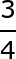In the fraction above, the 3 is the numerator because it appears on top.

So, if we are multiplying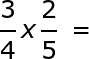We have to multiply the two numerators first. The numerators in this problem are 3 and 2 because they appear on top of the fractions.

3 x 2 = 6

## Denominator Times Denominator

After multiplying the numerators from left to right, the next step is to multiply the denominators of the fractions in the problem. The denominator is the number that appears at the bottom of a fraction.The 4 and 5 are the denominators in our equation because they appear at the bottom of each fraction.

4 x 5 = 20. So, the number 20 would represent the denominator of the final answer.

Our equation will look like this: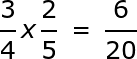## Simplify

The last step in multiplying fractions is to simplify, or break down your answer to lowest terms by reducing. To simplify a fraction, you must divide the top and bottom by the highest number that can divide into both numbers exactly.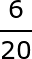This fraction can still be reduced because both the numerator and denominator can be divided evenly by two.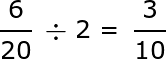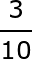This fraction is in lowest terms. (There is no number both 3 and 10 can be divided by evenly)

So the most exact answer for our problem is: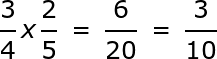## Examples of Multiplying Fractions

Let's practice with another problem.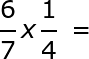Numerator x numerator. The two numerators are 6 and 1, so we will multiply 6 x 1 = 6.

Write 6 as the numerator for the answer.

Denominator x denominator. 7 and 4 are the denominators, so we will multiply 7 x 4 = 28.

Write 28 as the denominator in the answer.

Simplify -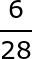This can be reduced or simplified by dividing the numerator and denominator by 2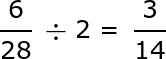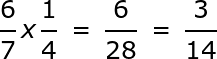To unlock this lesson you must be a Study.com Member.

### Register to view this lesson

Are you a student or a teacher?

#### See for yourself why 30 million people use Study.com

##### Become a Study.com member and start learning now.
Back
What teachers are saying about Study.com

### Earning College Credit

Did you know… We have over 200 college courses that prepare you to earn credit by exam that is accepted by over 1,500 colleges and universities. You can test out of the first two years of college and save thousands off your degree. Anyone can earn credit-by-exam regardless of age or education level.